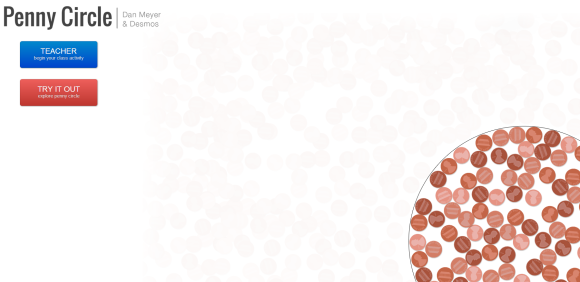• 10/10/13   |   Adjusted: 08/01/18   |   1 file# Penny Circle

Author: Dan Meyer and Desmos

• Description
• Files

Mathematically:

• Addresses standards: F-LE, F-IF.C.7,F-BF.B.3, A-CED.A.1, A-REI.B.4, and MP.4
• Requires students to confront the question of whether a model's predictions are reasonable (part of the modeling cycle)
• Sets up a situation with many avenues for mathematical investigation
• Uses technology efficiently to support the mathematics

In the classroom:

• Allows for student collaboration and discussion
• Provides a platform for the teacher to view students' work online
• Allows teacher to filter results, e.g., to see which students revised their models

This task was designed to include specific features that support access for all students and align to best practice for English Language Learner (ELL) instruction. Go here to learn more about the research behind these supports. This lesson aligns to ELL best practice in the following ways:

• Provides opportunities for students to practice and refine their use of mathematical language.
• Allows for whole class, small group, and paired discussion for the purpose of practicing with mathematical concepts and language.
• Includes a mathematical routine that reflects best practices to supporting ELLs in accessing mathematical concepts.
• Provides students with support in negotiating written word problems through multiple reads and/or multi-modal interactions with the problem.

• Making the Shifts

How does this task exemplify the instructional Shifts required by CCSSM?

 Focus Belongs to the Widely Applicable Prerequisites for College and Careers. Coherence Task helps to distinguish the concepts of linear, quadratic, and exponential growth Rigor Conceptual Understanding: secondary in this task Procedural Skill and Fluency: not targeted in this task Application: primary in this task

The task is hosted here. When you go to the page, click “Student Preview” to explore the task. Click  “Teacher Guide” to prepare for implementing the task.• Commentary

Penny Circle

This activity is a project from Dan Meyer and the Desmos Team

Goals

• Students will understand how to use math as a model, how to use smaller things to make predictions about bigger things.
• Students will understand the difference between linear, quadratic, and exponential models.

Getting Started

Share the link (labs.desmos.com/class/byige) with your students. Then look at the links on the left [of the teacher page] to explore their progress.

Time Required

45 minutes.

• Push that understanding around in productive ways.

• When it comes time to select a model - linear, exponential, or quadratic - make sure students fully understand the implications of that model and how it relates to their initial guess. If a student selects an exponential model, for example, say something like, "So your guess earlier was 500 pennies. The exponential model grows so fast it says the answer is millions of pennies. Either your earlier guess was way off or the exponential model isn't the right model. You decide which." Ask a similar question in the other direction if a student selects a linear model.
• Students need to understand WHY a quadratic is the best model here. It isn't enough to say that its prediction was closest to the actual answer. We have to know WHY it was closest. Ask for ideas. Ask them what happens to the area of a circle when the diameter goes from 3 inches to 6 inches. Some may say it doubles. If that's true, our penny's model should be linear. But it doesn't, it multiplies by 22. There's our quadratic model. (The formula for area of a circle also has a SQUARED term, which helps explain the model too.) One reason the exponential model is incorrect is that when you evaluate $y=ab^x$ for a zero inch circle, you get "a." In other words, the exponential model says a zero-inch circle has more than zero pennies in it. That can't happen.
• Ask students, "Did anybody change their model at all? Like from linear to quadratic or anything else. Why did you change?"
• Ask students to use their model in reverse. Ask them, "What if there were 2,000 pennies? What's the smallest circle that'd fit those pennies?"
• Ask them to think of other circumstances where this kind of modeling would be useful.
Another mathematical model for the "Pennies" problem could come from simply thinking about division. To estimate the number of pennies that fit in the circle, simply divide the area of the large circle, $\pi R^2$, by the area of a penny, $A_0$:
$$\left({\pi\over A_0}\right) \, R^2 \,.$$
The quadratic dependence emerges directly from this line of thinking. Students could also express the area of the penny as $\pi r^2$ and show that the model is quadratic in the dimensionless ratio $R/r$.